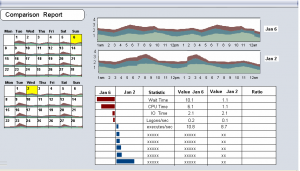## Redo over multiple weeks

June 30th, 2014

I’ve always wanted some sort of calendar view of load where I could see patterns across the same days of the week and same hours of the day and then be able to pick different periods and diff them:The first step of such a display would be selecting the data in such a way to represent the graphic. A graphic should be harder to do than a similar, though less powerful, ascii representation.

So here is a quick sketch of looking at redo generation over time. Right now I have to monitor how well redo can be archived which depends on how it fast it is generated. (typically what I’m more interested in is the general performance which is what the above graphic addressed)

The output below shows the redo generated per hour on average (picking the maximum hour in the bucket which is 4 hours long below) and the maximum generation at the minute level.  I may or may not be able to archive redo at the up to the minute but I do want to make sure I can keep up with each hour.

```DAY    HOUR  MAX(MB)    w1     w2      w3       w4       w5
SAT     0       9               5       4       4       3
SAT     4       12              4       5       4       4
SAT     8       1               0       0       0       0
SAT     12      0               0       0       0       0
SAT     16      1               0       0       0       0
SAT     20      4               1       0       0       1
SUN     0       10              3       3       1       3
SUN     4       13              6       5       3       5
SUN     8       7               6       6       0       6
SUN     12      7               0       3       0       4
SUN     16      1               0       0       1       0
SUN     20      8               3       2       0       3
MON     0       8               3       2       3       2
MON     4       7               2       3       2       1
MON     8       5               1       0       2       0
MON     12      1               0       0       1       0
MON     16      1               0       0       0       0
MON     20      7               2       2       0       2
TUE     0       14              6       5       7       4
TUE     4       7               1       1       1       2
TUE     8       3               0       0       0       0
TUE     12      1       1       0       0       0       0
TUE     16      1       1       0       0       0       0
TUE     20      3       1       1       1       1       1
WED     0       8       3       2       3       2       2
WED     4       7       2       1       3       2       2
WED     8       8       1       0       0       2       3
WED     12      7       1       0       0       1       1
WED     16      1       1       0       0       0       1
WED     20      4       1       1       1       1       1
THU     0       15      7       8       6       6       6
THU     4       8       2       1       1       1       1
THU     8       1       1       0       0       0       1
THU     12      16      1       11      0       0       1
THU     16      1       1       0       0       0       1
THU     20      4       1       1       1       1       1
FRI     0       11      2       2       2       2       2
FRI     4       8       3       1       1       1       1
FRI     8       4       1       0       0       0       0```
 `set` `heading ``off` `set` `feedback ``off` `set` `pagesize 0` `with` `pivot_data ``as` `(` `   ``select` `          ``WW pivot_col` `        ``, DY` `        ``, D` `        ``, HH` `        ``, ``max``(maxval) mv` `        ``, ``max``(average) av` `   ``from` `      ``( ``select` `distinct` `               ``begin_time,` `               ``4*trunc(to_char(begin_time,``'HH24'``)/4)     HH,` `               ``to_char(begin_time,``'DY'``)       DY,` `               ``mod(to_char(begin_time,``'D'``),7)  D,` `               ``mod(to_char(begin_time,``'WW'``),5)       WW,` `               ``average/(1024*1024) average,` `               ``maxval/(1024*1024) maxval,` `               ``snap_id` `        ``from`   `DBA_HIST_SYSMETRIC_SUMMARY` `        ``where`  `dbid=&&DBID ``and` `               ``metric_name=``'Redo Generated Per Sec'` `           ``and` `begin_time > sysdate - 31` `       ``)` `   ``group` `by` `HH,D,DY,WW` `)` `select` `DY ||``'   '``||` `       ``HH ||``'   '``||` `       ``round(``max``(mv),0)    ||``'  '``||` `       ``round(``max``(w1),0)  ||``'    '``||` `       ``round(``max``(w2),0)  ||``'    '``||` `       ``round(``max``(w3),0)  ||``'    '``||` `       ``round(``max``(w4),0)  ||``'    '``||` `       ``round(``max``(w5),0)` `from` `(` `   ``select` `*` `   ``from`  `pivot_data` `   ``pivot ( ``avg``(av)` `           ``for` `pivot_col ``in` `( 1 ``as` `w1,2 ``as` `w2,3 ``as` `w3 ,4 ``as` `w4 ,5 ``as` `w5 )` `         ``)` `)` `group` `by` `DY,D,HH` `order` `by` `D,HH` `/` `set` `heading ``on` `set` `feedback ``on` `set` `pagesize 30`

PS the above pivot is for 11g, for 10g here is the query without pivot

 `set` `pagesize 100` `col DY ``for` `A4` `col HH ``for` `99` `col mx ``for` `99` `col w1 ``for` `99` `col w2 ``for` `99` `col w3 ``for` `99` `col w4 ``for` `99` `col w5 ``for` `99` `with` `pivot_data ``as` `(` `   ``select` `          ``WW` `        ``, DY` `        ``, D` `        ``, HH` `        ``, ``max``(maxval) mv` `        ``, ``max``(average) av` `   ``from` `      ``( ``select` `distinct` `               ``begin_time,` `               ``4*trunc(to_char(begin_time,``'HH24'``)/4)     HH,` `               ``to_char(begin_time,``'DY'``)       DY,` `               ``mod(to_char(begin_time,``'D'``),7)  D,` `               ``mod(to_char(begin_time,``'WW'``),5)       WW,` `               ``average/(1024*1024) average,` `               ``maxval/(1024*1024) maxval,` `               ``snap_id` `        ``from`   `DBA_HIST_SYSMETRIC_SUMMARY` `        ``where` `               ``metric_name=``'Redo Generated Per Sec'` `           ``and` `begin_time > sysdate - 31` `       ``)` `   ``group` `by` `HH,D,DY,WW` `)` `select` `DY, HH,` `      ``round(``max``( mv ),0) mx,` `      ``round(``max``( decode( WW, 0 , av, ``null` `) ),0) w1,` `      ``round(``max``( decode( WW, 1 , av, ``null` `) ),0) w2,` `      ``round(``max``( decode( WW, 2 , av, ``null` `) ),0) w3,` `      ``round(``max``( decode( WW, 3 , av, ``null` `) ),0) w4,` `      ``round(``max``( decode( WW, 4 , av, ``null` `) ),0) w5` `   ``from`  `pivot_data` `group` `by` `DY,D,HH` `order` `by` `D,HH` `/`

Just to see the redo per hour can be done more simply as:

```set pagesize 100
col stat_name format a30
col MB format 9,999.99
select
btime, stat_name,
round((end_value-beg_value)/(1024*1024),2) MB
from (
select
e.stat_name,
to_char(s.BEGIN_INTERVAL_TIME,'DD-MON-YY HH24:MI')  btime,
e.value end_value,
Lag (e.value)
OVER( PARTITION BY e.stat_name ORDER BY s.snap_id) beg_value
from
DBA_HIST_SYSSTAT e,
DBA_HIST_SNAPSHOT s
where
s.snap_id=e.snap_id
and s.begin_interval_time > sysdate -2
and e.stat_name = 'redo size'
order by e.stat_name, begin_interval_time
)
where end_value-beg_value > 0
order by btime;```